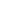### What is APY?

APY stands for annual percentage yield. It is the effective percentage amount earned on your deposit account, on a yearly basis. Depending on the balance in your account, and when you add or remove funds, this value can vary significantly.

According to the FDIC the general formula to be used to calculate the APY is as follows:
APY Earned (In Percentage terms) = 100*[(1 + Interest Earned/Balance)(365/Days in period) – 1]
“Interest Earned” is the actual interest earned on the account for the given time period (i.e. the month).
“Balance” is the average daily balance in the account over the time period.
“Days In period” is the actual days in the period (i.e. if the period is the month of January, this figure will always be 31).

NMLS ID: 2353898Equal Housing Lender Member FDIC © 2023 Northern Bank & Trust Company.